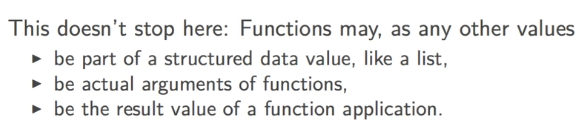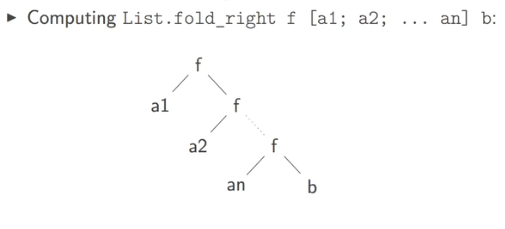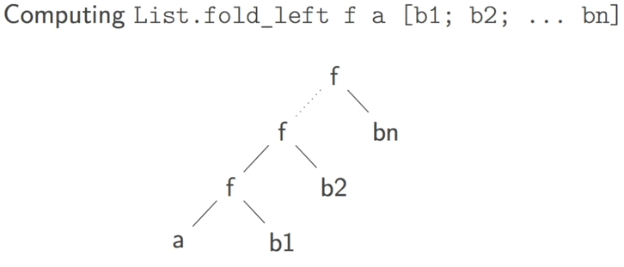# 1. FUNCTIONAL EXPRESSIONS

syntax for functional expr: `function some_identifier -> some_expr`
the type of the functional expr is `t1 -> t2` where t1 is the type of some_identifier, t2 is type of some_expr

ex.

```function x -> x + 1;;
(function x -> 2*x) 5;; (*annonymous function*)
```

the previous way of defining function:

`let f x = e`,

is just an abbreviation for common let-binding:

`let f = function x -> e`

In fact, the most general form of a function definitoin contains a seq of pattern matching:

```function
| pattern_1 -> expr_1
| pattern_2 -> expr_2
```

When only 1 pattern, the `|` is omitted.

example

```let rc length = function
| [] -> 0
| _::r -> 1 + length r;;
type expr =
| Int of int
| Add of expr * expr;;
let rec eval = function
| Int n -> n
| Add(e1, e2) -> (eval e1) + (eval e2);;
```

# 2. FUNCTIONS AS FIRST-CLASS VALUES

functional types are just values of a particular type, thus this allows the uniform way of naming a value let x = ...

Types govern function application. We can apply e1 to e2 when:

• e1 has type t1 -> t2
• t1 matchs the type of e2```let f1 = [(function x -> x+1); (function x -> 2*x)];;
(List.hd fl) 17;;

let apply_twice f x = f (f x);;
apply_twice (function x -> 2*x) 1;;
let rec apply_n_times f n x =
if n<=0 then x
else apply_n_times f (n-1) (f x);;
apply_n_times (function x -> 2*x) 10 1;;

let compose f g = (function x -> f (g x));;
let fg = compose (function x->x+1) (function x->2*x) in
fg 10;;
```

### function pitfalls

function apply from left to right (function application associates to the left):

`exp1 exp2 exp3`
is equivalent to: `(exp1 exp2) exp3`

```let double = function x -> 2*x;;
double double 5;;
double (double 5);;
```

# 3. FUNCTIONS WITH MULTIPLE ARGUMENTS

anonymous function with several arguments: use keyword `fun`:
`fun p1 ... pn -> exp`

unlike `function` keyword, `fun` only admits one case/branch

remark: funs with several arguments are just abbrevations for single-argument functions that returns a function:

```let f1 = function n -> (function x -> n+x);;
(f1 17) 73;;
f1 17 73;;
let f2 = fun n x -> n+x;;
f2 17 73;;
(f2 17) 73;;
```

in fact, `fun x1 ... xn -> e` is just abbreviation for: `function x1 -> (..(function x2 -> ... -> (function xn -> e)..)`

# 4. PARTIAL FUNCTION APPLICATION

`let f = fun x y -> exp` is equivalent to: `let f = function x -> (function y -> exp)`

⇒ partially apply f (ie, `f x`) will give a function.

```let f1 = fun x y x -> x + y *z;;
let f2 = f1 1;;
let f3 = f2 2;;
f3 4;;
```

what happens at func-application:
when applying `f = function x->e` to `a`:
→ evaluate `e` in the context `x=a`
→ the arrow `->` will block any evaluation

```let f = fun x y -> (x / 0) + y;;
let f2 = f1 17;; (*error will not happen here, as the `->` blocks the evaluation!*)
f2 42;;
```

### partial evaluation

sometimes we can do part of a calculation as soon as we have the frist few arguments
⇒ extract that part of calculation before the arrow !

```let egal l1 l2 =
List.sort compare l1 = List.sort compare l2;;
let egalp l1 =
let l1sorted = List.sort compare l1 in (*sort the 1st argument before going to the next functional abstraction*)
function l2 -> l1sorted = List.sort compare l2;
```

# 5. MAPPING FUNCTIONS ON LISTS

many useful functions in the `List` module, either `open List` at beginning, or with pointed notation (`List.hd`)

### implementation

```let rec map f = function
| [] -> []
| h::r -> (f h):(map f r);;
map (function x -> x*x) [1;2;3;4;5];;

let map2 f l1 l2 = match (l1, l2) with
| [],[] -> []
| h1::r1, h2::r2 -> (f h1 h2)::(map2 f r1 r2)
| _ -> raise (Invalid_argument "List.map2");;
map2 (fun x y -> x+y) [1;2;3] [10;20;30];;
```

### examples

example1: int vectors/matrices

row vector: int list
matrix: list of row vectors
turn infix operators into functions : using parentheses `(+) (/) ( * )` ...(note: spaces are necessary for `*`, otherwise this turns into comments...

```let vsum  = List.map2 (+);; (* use partial application *)
vsum [1;2;3] [10;20;30];;
let msum = List.map2 (List.map2 (+));; (* nested partial application *)
msum [[1;2;]; [3;4]] [[10;20]; [30;40]];;
```

example2: get all sublists of a list
type: `a' list -> 'a list list`
write using induction:

```let rec sublists = function
| [] -> [ [] ]
| h::r ->
let rp = sublists r
let appendh = function lst -> h::lst in
rp @ (map appendh rp)
```

# 6. FOLDING FUNCTIONS ON LISTS

• map: apping a unary function on list, all elements considered isolated.
• folding: combining all elements of a list using a binary operator.
• 2 different ways of folding: fold-left/fold-right

### fold_right

`fold_right: ('a -> 'b -> 'b) -> 'a list -> 'b -> 'b`implementation and usage:

```let rec fold_right f l b = match l with
| [] -> b
| h::r ->  f h (fold_right f r b);;
fold_right (+) [1;2;3;4] 0;;
let concat = fold_right (fun x y -> x::y);; (partial application of fold_right)
concat [1;2;3] [3;4;5];;
```

### fold_left

`fold_left: ('a -> 'b -> 'a) -> 'a -> 'b list -> 'a`
note: the default value's position is different from that of fold_right !```let rec fold_left f a l = match l with
| [] -> a
| h::r -> fold_left f (f a h) r;;
fold_left (+) 0 [1;2;3];;
let reverse = fold_left (fun x y -> y::x) [];; (* partial application *)
reverse [1;2;3;4];;
```

### examples

example1: Inner product of int vectors
first get pairwise product, then sum up.

```let product v1 v2 = List.fold_left (+) 0 (List.map2 ( * ) v1 v2);;
product [1;2;3] [4;5;6];;
```

example2: countif

```let countif p l = List.fold_left
(fun acc elem -> if p elem then acc+1 else acc) 0 l;;
countif (fun x-> x>0) [3;1;-2;0;4];;
```